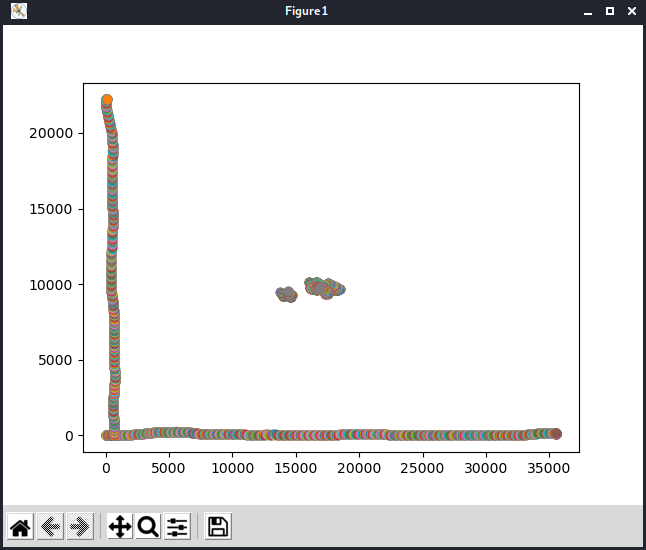# Crystal from the past

## Description

``````- Hey, Nux! Look at this crystal! It's almost as nice as a V8 engine!
- What?
- It looks like... a chrome... thingy.
- What are you talking about. Let me see.
- NO! ITS MINE! I... MUST... DRAW!
``````

One of Nux’s weirdo friend found a mesmerizing crystal in the desert. After taking it with him, he got posessed with it. He tells weird jokes no one understands, and draws a lot on his shitty computer using an unrecognizeable graphic tablet as old as mankind. However, he won’t let you see the drawings, thus, you installed a packet sniffer on his computer.

You managed to get a pcap of one of his drawing. He starts every session by calibrating the graphic tablet. This is achieved by drawing a horizontal and a vertical line, pushing the tip of the pen (draw force calibration), and tilting the pen around.

## Solution

I started this challenge with looking at the given `calibration.pcap` file inside Wireshark. I noticed the `USB descriptor` but since google didn’t gave me an understandable protocol description easily, I tried to understand the sent bytes in a logical manner. We knew the following from the description:

• Our guy starts with a horizontal line
• Followed by a vertical line
• Then some other calibration stuff

This meant to me, I am looking for an `x` and a `y` variable sent, signaling the pen’s position, and some other stuff, which might be handy later, e.g. `force` and `tilt`. I exported the captured data from Wireshark, and started working. (Yes I knew about PyShark, but I couldn’t make it to work, since it failed to load the data from me from the packets.) This is the script I came up with, which loads the data, and then plots it to a diagram.

``````#!/bin/env python3
import numpy as np
import matplotlib.pyplot as plt

file = open('./calibration.data', 'r')
#file = open('./chall.data', 'r')

data = []

if ('02' in line):
raw = line.strip('\n')
cut = [raw[i+2:i+4] + raw[i:i+2] for i in range(0, len(raw), 4)]
d = {
'idk': int('0x' + cut,16),
'x': int('0x' + cut,16),
'y': int('0x' + cut,16),
'force': int('0x' + cut,16),
'tilt': int('0x' + cut,16)
}
data.append(d)

#Plot the data
for item in data:
print(item)
plt.scatter(item['x'], item['y'])

plt.show()
``````From here I started working with the real data, however on the first render it looked quite a mess. Of course, up until now I only used the `x` and `y` variables, so I figured I would tinker a little bit with the other values. This setup gave me a clear enough diagram, so I could read the flag:

``````for item in data:
print(item)
if item['x'] < 14700 and item['y'] < 9700:
if item['tilt'] > 200 and item['force'] > 0:
plt.scatter(item['x'], item['y'])
``````

The acquired flag is:

``````cd21{HIDDEN}
``````

← Back to SecChallenge21